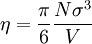# Packing fraction$\eta = \frac{ \pi }{6} \frac{ N \sigma^3 }{V}$
where$\sigma$ is the hard sphere diameter.